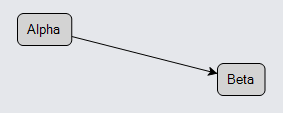It is common to add annotations or decorations on a link, particularly text.

By default if you add a GraphObject to a Link, it will be positioned at the middle of the link. In this example, we just add a TextBlock to the link and bind its TextBlock.Text property to the link data's "Text" property.

``````
diagram.NodeTemplate =
new Node("Auto")
.Bind("Location", "Loc", Point.Parse)
new Shape("RoundedRectangle") { Fill = "lightgray" },
new TextBlock { Margin = 5 }
.Bind("Text", "Key")
);

new Shape(),  // this is the link shape (the line)
new Shape { ToArrow = "Standard" },  // this is an arrowhead
new TextBlock().Bind("Text")  // this is a Link label
);

diagram.Model =
new MyModel {
NodeDataSource = new List<NodeData> {
new NodeData { Key = "Alpha", Loc = "0 0" },
new NodeData { Key = "Beta", Loc = "200 50" }
},
new LinkData { From = "Alpha", To = "Beta", Text = "a label" }
}
};
``````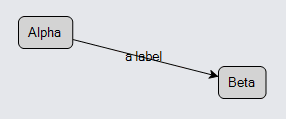Note that clicking on the text label results in selection of the whole Link.

Although it is commonplace to use a TextBlock as the link label, it can be any GraphObject such as a Shape or an arbitrarily complex Panel. Here is a simple Panel label:

``````
diagram.NodeTemplate =
new Node("Auto")
.Bind("Location", "Loc", Point.Parse)
new Shape("RoundedRectangle") { Fill = "lightgray" },
new TextBlock { Margin = 5 }
.Bind("Text", "Key")
);

new Shape(),
new Shape { ToArrow = "Standard" },
new Panel("Auto")  // this whole Panel is a link label
new Shape("Ellipse") { Fill = "yellow", Stroke = "gray" },
new TextBlock { Margin = 3 }
.Bind("Text")
)
);

diagram.Model =
new MyModel {
NodeDataSource = new List<NodeData> {
new NodeData { Key = "Alpha", Loc = "0 0" },
new NodeData { Key = "Beta", Loc = "200 50" }
},
new LinkData { From = "Alpha", To = "Beta", Text = "hello!" }
}
};
``````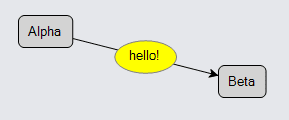This also works if the link is orthogonally routed or bezier-curved.

``````
diagram.NodeTemplate =
new Node("Auto")
.Bind("Location", "Loc", Point.Parse)
new Shape("RoundedRectangle") { Fill = "lightgray" },
new TextBlock { Margin = 5 }
.Bind("Text", "Key")
);

new Shape(),
new Shape { ToArrow = "Standard" },
new TextBlock { TextAlign = TextAlign.Center }  // centered multi-line text
.Bind("Text")  // this is a Link label
);

diagram.Model =
new MyModel {
NodeDataSource = new List<NodeData> {
new NodeData { Key = "Alpha", Loc = "0 0" },
new NodeData { Key = "Beta", Loc = "200 50" }
},
new LinkData { From = "Alpha", To = "Beta", Text = "a label\non an\northo link" }
}
};
``````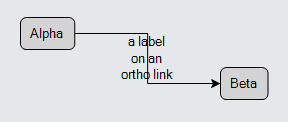Although positioning the label at the middle of the link is the default behavior, you can set GraphObject properties that start with "Segment" to specify exactly where and how to arrange the object along the route of the link.

## Link label SegmentIndex and SegmentFraction

Set the GraphObject.SegmentIndex property in order to specify which segment of the link route the object should be on. Set the GraphObject.SegmentFraction property to control how far the object should be, as a fraction from the start of the segment (zero) to the end of the segment (one).

When setting the GraphObject.SegmentIndex property to NaN, the fraction will be calculated along the entire link route instead of a particular segment.

In the case of a link that comes from a node with no GraphObject.FromSpot (i.e. Spot.None) and goes to a node with no GraphObject.ToSpot, there may be only one segment in the link, segment number zero.

``````
diagram.NodeTemplate =
new Node("Auto")
.Bind("Location", "Loc", Point.Parse)
new Shape("RoundedRectangle") { Fill = "lightgray" },
new TextBlock { Margin = 5 }
.Bind("Text", "Key")
);

new Shape(),
new Shape { ToArrow = "Standard" },
new TextBlock("from") { SegmentIndex = 0, SegmentFraction = 0.2 },
new TextBlock("mid") { SegmentIndex = 0, SegmentFraction = 0.5 },
new TextBlock("to") { SegmentIndex = 0, SegmentFraction = 0.8 }
);

diagram.Model =
new MyModel {
NodeDataSource = new List<NodeData> {
new NodeData { Key = "Alpha", Loc = "0 0" },
new NodeData { Key = "Beta", Loc = "200 50" }
},
new LinkData { From = "Alpha", To = "Beta" }
}
};
``````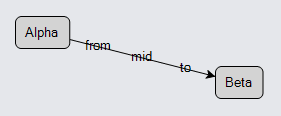In the case of a link that has many segments in it, you will want to specify different segment numbers. Orthogonal links, for example, typically have 6 points in the route, which means five segments numbered from 0 to 4.

``````
diagram.NodeTemplate =
new Node("Auto")
.Bind("Location", "Loc", Point.Parse)
new Shape("RoundedRectangle") { Fill = "lightgray" },
new TextBlock { Margin = 5 }
.Bind("Text", "Key")
);

new Shape(),
new Shape { ToArrow = "Standard" },
new TextBlock("from") { SegmentIndex = 1, SegmentFraction = 0.5 },
new TextBlock("mid") { SegmentIndex = 2, SegmentFraction = 0.5 },
new TextBlock("to") { SegmentIndex = 3, SegmentFraction = 0.5 }
);

diagram.Model =
new MyModel {
NodeDataSource = new List<NodeData> {
new NodeData { Key = "Alpha", Loc = "0 0" },
new NodeData { Key = "Beta", Loc = "200 50" }
},
new LinkData { From = "Alpha", To = "Beta" }
}
};
``````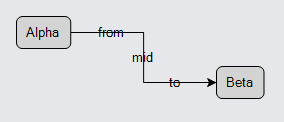However, you can also count segments backwards from the "to" end of the link. -1 is the last segment, -2 is the next to last, etc. When you use a negative segment index, the segment fraction goes from 0 closest to the "to" end to 1 for the end of that segment that is farthest back along the route from the "to" end. Thus a SegmentIndex of -1 with a SegmentFraction of 0 is the very end point of the link route. A SegmentIndex of -1 with a SegmentFraction of 1 is the same point as SegmentIndex -2 and SegmentFraction 0.

For labels that belong near the "to" end of a link, you will normally use negative values for GraphObject.SegmentIndex. This convention works better when the number of segments in a link is unknown or may vary.

Lastly, one can specify a SegmentIndex of NaN to have the fraction calculated along the entire link route instead of just a particular segment.

``````
diagram.NodeTemplate =
new Node("Auto")
.Bind("Location", "Loc", Point.Parse)
new Shape("RoundedRectangle") { Fill = "lightgray" },
new TextBlock { Margin = 5 }
.Bind("Text", "Key")
);

new Shape(),
new Shape { ToArrow = "Standard" },
new TextBlock("1/3") { SegmentIndex = double.NaN, SegmentFraction = 0.33 },  // label at 1/3 of link length
new TextBlock("2/3") { SegmentIndex = double.NaN, SegmentFraction = 0.67 }  // label at 1/3 of link length
);

diagram.Model =
new MyModel {
NodeDataSource = new List<NodeData> {
new NodeData { Key = "Alpha", Loc = "0 0" },
new NodeData { Key = "Beta", Loc = "200 50" }
},
new LinkData { From = "Alpha", To = "Beta" }
}
};
``````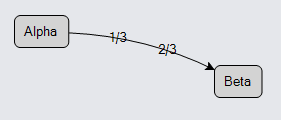## Link label SegmentOffset and AlignmentFocus

There are two ways of making small adjustments to the position of a label object given a particular point on a link segment specified by the segment index and fractional distance.

The GraphObject.SegmentOffset property controls where to position the object relative to the point on a link segment determined by the GraphObject.SegmentIndex and GraphObject.SegmentFraction properties. The offset is not a simple offset of the point -- it is rotated according to the angle of that link segment. A positive value for the Y offset moves the label element towards the right side of the link, as seen going in the direction of the link. Naturally a negative value for the Y offset moves it towards the left side.

``````
diagram.NodeTemplate =
new Node("Auto")
.Bind("Location", "Loc", Point.Parse)
new Shape("RoundedRectangle") { Fill = "lightgray" },
new TextBlock { Margin = 5 }
.Bind("Text", "Key")
);

new Shape(),
new Shape { ToArrow = "Standard" },
new TextBlock("left") { SegmentOffset = new Point(0, -10) },
new TextBlock("right") { SegmentOffset = new Point(0, 10) }
);

diagram.Model =
new MyModel {
NodeDataSource = new List<NodeData> {
new NodeData { Key = "Alpha", Loc = "0 0" },
new NodeData { Key = "Beta", Loc = "200 50" }
},
new LinkData { From = "Alpha", To = "Beta" }
}
};
``````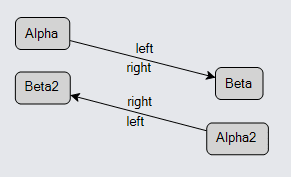Another way to change the effective offset is by changing the spot in the object that is being positioned relative to the link segment point. You can do that by setting the GraphObject.AlignmentFocus, which as you have seen above defaults to Spot.Center. (GraphObject.AlignmentFocus is also used by other Panel types, which is why its name does not start with "Segment".)

``````
diagram.NodeTemplate =
new Node("Auto")
.Bind("Location", "Loc", Point.Parse)
new Shape("RoundedRectangle") { Fill = "lightgray" },
new TextBlock { Margin = 5 }
.Bind("Text", "Key")
);

new Shape(),
new Shape { ToArrow = "Standard" },
new TextBlock("left") { AlignmentFocus = new Spot(1, 0.5, 3, 0) },
new TextBlock("right") { AlignmentFocus = new Spot(0, 0.5, -3, 0) }
);

diagram.Model =
new MyModel {
NodeDataSource = new List<NodeData> {
new NodeData { Key = "Alpha", Loc = "0 0" },
new NodeData { Key = "Beta", Loc = "200 50" }
},
new LinkData { From = "Alpha", To = "Beta" }
}
};
``````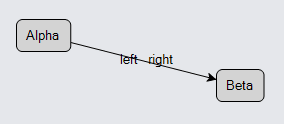Yet you may instead want to control the angle of the individual labels based on the angle of the link segment.

The GraphObject.SegmentOrientation property controls the angle of the label object relative to the angle of the link segment. There are several possible values that you can use. The default orientation is Orientation.None, meaning no rotation at all. Orientation.Along is commonly used to have the object always rotated at the same angle as the link segment. Orientation.Upright is like "Along", but is often used when there is text in the label, to make it easier to read.

``````
diagram.NodeTemplate =
new Node("Auto")
.Bind("Location", "Loc", Point.Parse)
new Shape("RoundedRectangle") { Fill = "lightgray" },
new TextBlock { Margin = 5 }
.Bind("Text", "Key")
);

new Shape(),
new Shape { ToArrow = "Standard" },
new TextBlock("left") { SegmentOffset = new Point(0, -10), SegmentOrientation = Orientation.Upright },
new TextBlock("middle") { SegmentOffset = new Point(0, 0), SegmentOrientation = Orientation.Upright },
new TextBlock("right") { SegmentOffset = new Point(0, 10), SegmentOrientation = Orientation.Upright }
);

diagram.Model =
new MyModel {
NodeDataSource = new List<NodeData> {
new NodeData { Key = "Alpha", Loc = "0 0" },
new NodeData { Key = "Beta", Loc = "200 50" }
},
new LinkData { From = "Alpha", To = "Beta" }
}
};
``````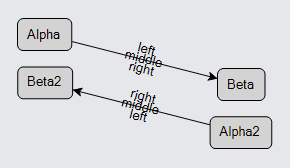Now if you move a node around you will always be able to read the label texts, and yet each label stays on its intended side of the link, as seen going in the direction of the link.

This points out a difference between a SegmentIndex/SegmentFraction pair of 0/1 and 1/0. Although they both refer to the same point, the angle associated with the first pair is the angle of the first segment (segment 0), whereas the angle associated with the second pair is the angle of the second segment.

## Link labels near the ends

For labels that are near either end of a link, it may be convenient to set the GraphObject.SegmentOffset to Point(NaN, NaN). This causes the offset to be half the width and half the height of the label object.

``````
diagram.NodeTemplate =
new Node("Auto")
.Bind("Location", "Loc", Point.Parse)
new Shape("RoundedRectangle") { Fill = "lightgray" },
new TextBlock { Margin = 5 }
.Bind("Text", "Key")
);

new Shape(),
new Shape { ToArrow = "Standard" },
new TextBlock("from") {
SegmentIndex = 0, SegmentOffset = new Point(double.NaN, double.NaN),
SegmentOrientation = Orientation.Upright
},
new TextBlock("to") {
SegmentIndex = -1, SegmentOffset = new Point(double.NaN, double.NaN),
SegmentOrientation = Orientation.Upright
}
);

diagram.Model =
new MyModel {
NodeDataSource = new List<NodeData> {
new NodeData { Key = "Alpha", Loc = "0 0" },
new NodeData { Key = "Beta", Loc = "200 50" }
},
new LinkData { From = "Alpha", To = "Beta" }
}
};
``````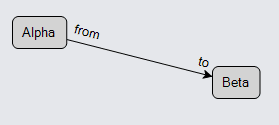Now that you know more about the GraphObject "Segment..." properties for controlling the position and angle of objects in a Link, it is easy to explain how arrowheads are defined. Arrowheads are just labels: Shapes that are initialized in a convenient manner.

You can see a copy of all of the built-in arrowhead definitions in this file: Arrowheads.cs.

Here are the equivalent settings for initializing an arrowhead Shape by setting Shape.ToArrow to "Standard".

``````
diagram.NodeTemplate =
new Node("Auto")
.Bind("Location", "Loc", Point.Parse)
new Shape("RoundedRectangle") { Fill = "lightgray" },
new TextBlock { Margin = 5 }
.Bind("Text", "Key")
);

new Shape(),
new Shape {
// the following are the same as { ToArrow = "Standard" }:
SegmentIndex = -1,
SegmentOrientation = Orientation.Along,
AlignmentFocus = Spot.Right,
Geometry = Geometry.Parse("F1 m0 0 l8 4  -8 4  2 -4 z")
}
);

diagram.Model =
new MyModel {
NodeDataSource = new List<NodeData> {
new NodeData { Key = "Alpha", Loc = "0 0" },
new NodeData { Key = "Beta", Loc = "200 50" }
},